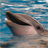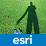# Convert a lat long value to ESRI Map Point in ArcGis using Java

12643
6
09-11-2012 06:47 PMNew Contributor
Hi Experts,

I have the latitude and longitude values of a particular building. I am developing an Android application using ESRI maps. Below is the extent of the map.

Initial Extent:
XMin: -8566095.96034392
YMin: 4719176.71718385
XMax: -8564317.60510787
YMax: 4720265.94483682
Spatial Reference: 102100 (3857)

Full Extent:
XMin: -8569852.61240501
YMin: 4715537.21773259
XMax: -8559507.60286413
YMax: 4724195.31894739
Spatial Reference: 102100 (3857)

Units: esriMeters

I am facing the following difficulties.
1) Describing a point in Java using lat and long value.
2)Converting the point from lat long form to the co-ordinate system in which the above details are.
Tags (2)
6 RepliesOccasional Contributor

Hi,

A Point (com.esri.core.geometry.Point) in the API consists of an x coordinate and a y coordinate, so to create a point from lat and lon values, you can do:

Point point = new Point(lon, lat); // x is longitude and y is latitude)

So far the Point doesn't care about the spatial reference. When you come to add it to a map, however, you need to make sure your Point has x and y values in the correct units/spatial reference, i.e. the ones of your map. Lat-lon coordinates describe points in the 4326 or WGS84 spatial reference, and you want to project the points to the 102100 spatial reference.

We have methods in the GeometryEngine class to project geometries (such as Points) from one spatial reference to another. One is project(double x, double y, SpatialReference sr) and it takes lon/lat coordinates, and returns a point in the specified spatial reference.

So in your case you can do something like:

Point projectedPoint = GeometryEngine.project(lon, lat, SpatialReference.create(102100));

or more generally, just use the spatial reference of your map:

Point projectedPoint = GeometryEngine.project(lon, lat, map.getSpatialReference());

~eliseNew Contributor

how i do the same with a polyline?

geometry returns a point? who i get the correct x,y values for polyline path?Occasional Contributor

You can use another overload of the 'project' method I link to in my previous comment. For example the next one below takes a Geometry as a parameter, an input spatial reference, and an output spatial reference. It returns a geometry, so if you pass in a polyline you'll get a polyline back and so on. The output spatial reference you can get from your map. The input spatial reference if you're using lat lon points will be WGS84 with wkid=4326, so you can create a SpatialReference object, to use as input spatial reference in these conversions, like this: SpatialReference.create(4326).

~eliseNew Contributor

i dont understant how to implement this in code?

(23.63733,37.94721);

(24.63733,38.94721);

i have a code :

Polyline lineGeometry = new Polyline();

lineGeometry.startPath(23.63733,37.94721);

lineGeometry.lineTo(24.63733,38.94721);

but it not shown at correct place.

for point in this coordinates i use this code and it work like a charm on my map

Point pointGeometry = GeometryEngine.project(23.63733,37.94721, SpatialReference.create(102113));

Point pointGeometry = GeometryEngine.project(24.63733,38.94721, SpatialReference.create(102113));

how to use this with a polyline;New Contributor

ok i got it.

i use:

lineGeometry.startPath( GeometryEngine.project(23.63733,37.94721, SpatialReference.create(102113)));

lineGeometry.lineTo(GeometryEngine.project(24.07617,35.49069, SpatialReference.create(102113)));

and it worked like a charm!Occasional Contributor

I suggest you try using this project method overload.

You can project your polyline in one go:

// spatial reference object you can reuse

SpatialReference wgs84 = SpatialReference.create(4326);

// project geometry and cast to the correct output geometry

Polyline projectedPolyline = (Polyline) GeometryEngine.project(lineGeometry, wgs84, map.getSpatialReference());

There's other ways of doing it, and your code above works as you say, but if you do this lots of time you'll probably want to avoid projecting each point individually and avoid creating a SpatialReference object each time.

~elise